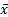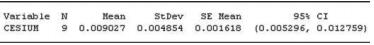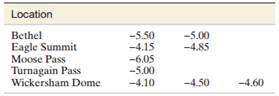### Create an Account

Already have account?

### Forgot Your Password ?

Home / Questions / Radioactive lichen Refer to the Alaskan Lichen Radionuclide Baseline Research study prese...

# Radioactive lichen Refer to the Alaskan Lichen Radionuclide Baseline Research study presented in Exercise 7 39 p 318 In a sample of n 9 lichen specimens the researchers found the mean and

Radioactive lichen. Refer to the Alaskan Lichen Radionuclide Baseline Research study, presented in Exercise 7.39 (p. 318). In a sample of n = 9 lichen specimens, the researchers found the mean and standard deviation of the amount of the radioactive element, cesium-137, that was present to be .009 and .005 microcurie per milliliter, respectively. Suppose the researchers want to increase the sample size in order to estimate the mean μ to within .001 microcurie per milliliter of its true value, using a 95% confidence interval.

a. What is the confidence level desired by the researchers?

b. What is the sampling error desired by the researchers? c. Compute the sample size necessary to obtain the desired estimate.

Exercise 7.39

Radioactive lichen. Refer to the Lichen Radionuclide Baseline Research project at the University of Alaska, presented in Exercise 2.36 (p. 47). Recall that the researchers collected 9 lichen specimens and measured the amount (in microcuries per milliliter) of the radioactive element cesium-137 for each. (The natural logarithms of the data values are saved in the LICHEN file.) A MINITAB printout with summary statistics for the actual data is shown below.

a. Give a point estimate for the mean amount of cesium in lichen specimens collected in Alaska.

b. Give the t -value used in a small-sample 95% confidence interval for the true mean amount of cesium in Alaskan lichen specimens.

c. Use the result you obtained in part b and the values ofand s shown on the MINITAB printout to form a 95% confidence interval for the true mean amount of cesium in Alaskan lichen specimens.

d. Check the interval you found in part c with the 95% confidence interval shown on the MINITAB printout.

e. Give a practical interpretation for the interval you obtained in part c.Exercise 2.36

Radioactive lichen. Lichen has a high absorbance capacity for radiation fallout from nuclear accidents. Since lichen is a major food source for Alaskan caribou, and caribou are, in turn, a major food source for many Alaskan villagers, it is important to monitor the level of radioactivity in lichen. Researchers at the University of Alaska, Fairbanks, collected data on nine lichen specimens at various locations for this purpose. The amount of the radioactive element cesium-137 was measured (in microcuries per milliliter) for each specimen. These data are saved in the LICHEN file. The data values, converted to logarithms, are given in the following table (note that the closer the value is to zero, the greater is the amount of cesium in the specimen).a. Construct a dot plot for the nine measurements.

b. Construct a stem-and-leaf display for the nine measurements.

c. Construct a histogram plot of the nine measurements.

d. Which of the three graphs in parts a–c, respectively, is most informative?

e. What proportion of the measurements has a radioactivity level of - 5.00 or lower?

Aug 07 2020 View more View Less

#### Answer (Solved)Subscribe To Get Solution